Defining trigonometry:

When we hear this word, various angles and ratios start circling around our head. It is indeed valid, as “Trigono” means three and “metry” means measurement. So trigonometry is all about measurement of sides and angles of a three-sided figure – The triangle.

Let’s start our discussion by talking about right-angled triangle. We have names for the sides of such triangle. The longest one is called Hypotenuse and the other two sides are called base and opposite side (relative to an angle). So, in ΔABC, if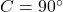, then angle opposite to A is called opposite side and the one adjacent to it is called Base. I’ve talked about this in the video.

The ratios

This triangle helps us to define the trigonometric ratios about which the complete trigonometry moves around. I will name all here with their abbreviations in bracket :

Sine(Sin)

Cosine(Cos)

Tangent(Tan)

Cosecant(Csc/Cosec)

Secant(Sec)

Cotangent(Cot).

So, in triangle right-angled ΔABC, if, then :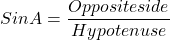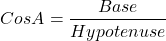Wait. Where are the other three? So once we know above, the others are pretty straight forward: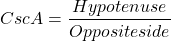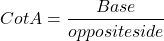Basic identities:

From above, we just noticed three identities: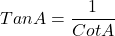Three more basic identities which will make life easy while moving ahead: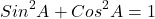Standard angles:

There are a few angles at which we must remember the values of above 6 ratios. They are :I have mentioned the trigonometric values in the video.

So,.  But what isAngle can not only be represented in degrees, but in radians as well.

The relation between degrees and radians is given by :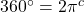Yes radians is denoted with a c

Following are the quick conversions:Here are some worked out examples to have a better understanding: